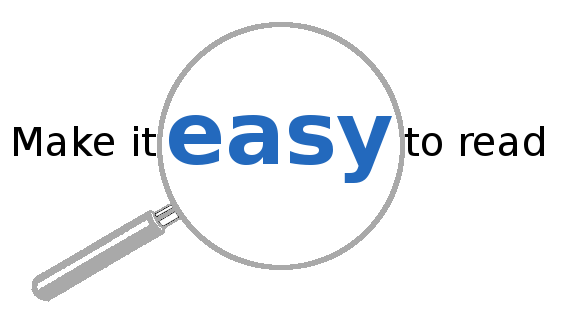# Readable code with base R (part 1)

Producing readable R code is of great importance, especially if there is a chance that you will share your code with people other than your future self. In this series of blog posts, I will present some (often underused) base R functions for this purpose.In this post, we cover `startsWith`, `endsWith`, and `Filter`.

### `startsWith` and `endsWith` for string-matching

There are special base functions for pre- or postfix matching.

``````# Basic usage:
w <- "Hello World!"
startsWith(w, "Hell")
``````
`` TRUE``
``````startsWith(w, "Helo")
``````
`` FALSE``
``````endsWith(w, "!")
``````
`` TRUE``

Of course, it also works with vectors. Can’t remember the exact name of a base function? Try this… ;)

``````base_funcs <- ls("package:base")

base_funcs[startsWith(base_funcs, "row")]
``````
``````  "row"                    "row.names"
 "row.names.data.frame"   "row.names.default"
 "row.names<-"            "row.names<-.data.frame"
 "row.names<-.default"    "rowMeans"
 "rownames"               "rownames<-"
 "rowsum"                 "rowsum.data.frame"
 "rowsum.default"         "rowSums"               ``````

The ‘readable’ property really shines when combined with control-flow.

``````tell_file_type <- function(fn) {
# Check different file endings
if (endsWith(fn, "txt")) {
print("A text file.")
}
if (any(endsWith(fn, c("xlsx", "xls")))) {
print("An Excel file.")
}
}
tell_file_type("A.txt")
``````
`` "A text file."``
``````tell_file_type("B.xls")
``````
`` "An Excel file."``

The resulting code reads very well.

### `Filter`

Using another nice base function, `Filter`, the above code can be further improved.

``````get_file_type <- function(fn) {
file_endings <- c(text="txt", Excel="xls", Excel="xlsx")
Filter(file_endings, f = function(x) endsWith(fn, x))
}

get_file_type("C.xlsx")
``````
`````` Excel
"xlsx" ``````

Again, very readable to my eyes. It should be noted that for this particular problem using `tools::file_ext` is even more appropriate, but I think the point has been made.

Last but not least, since `Filter` works on lists, you can use it on a `data.frame` as well.

``````dat <- data.frame(A=1:3, B=5:3, L=letters[1:3])
dat
``````
``````  A B L
1 1 5 a
2 2 4 b
3 3 3 c``````
``````Filter(dat, f = is.numeric)
``````
``````  A B
1 1 5
2 2 4
3 3 3``````
``````Filter(dat, f = Negate(is.numeric))  # or Filter(dat, f = function(x) !is.numeric(x))
``````
``````  L
1 a
2 b
3 c``````

That’s it for now - see you in part 2.

### Reuse

Text and figures are licensed under Creative Commons Attribution CC BY-SA 4.0. The figures that have been reused from other sources don't fall under this license and can be recognized by a note in their caption: "Figure from ...".